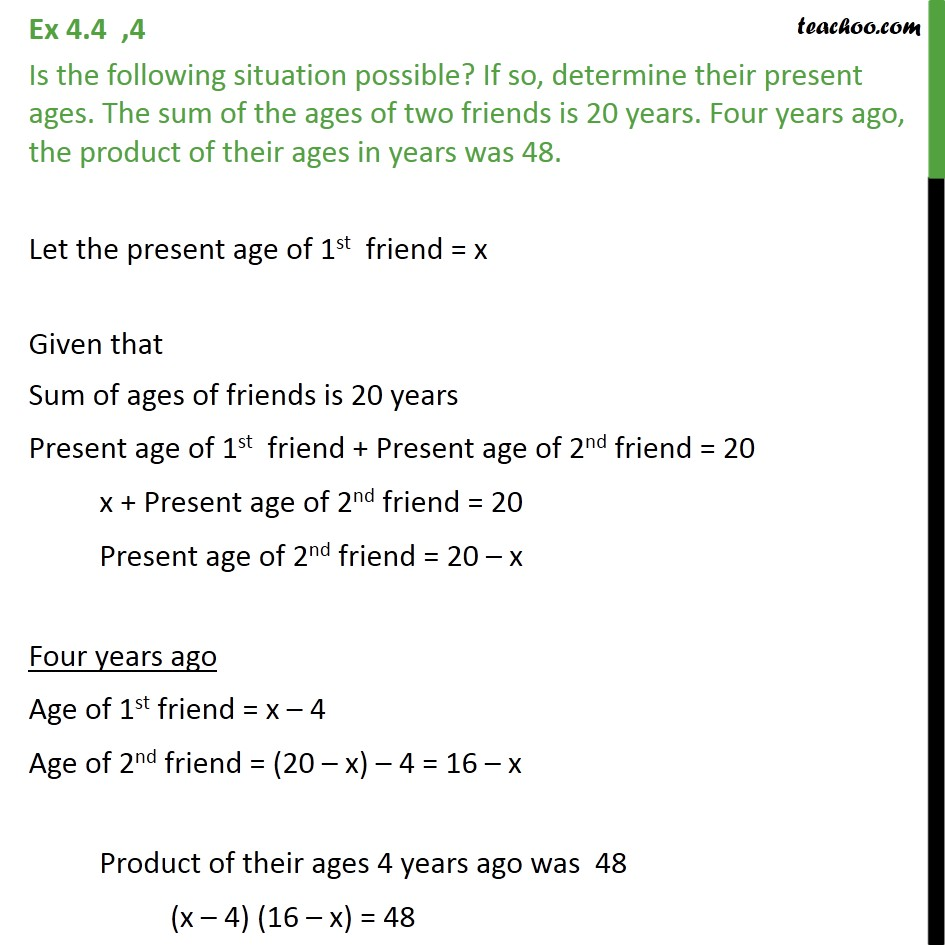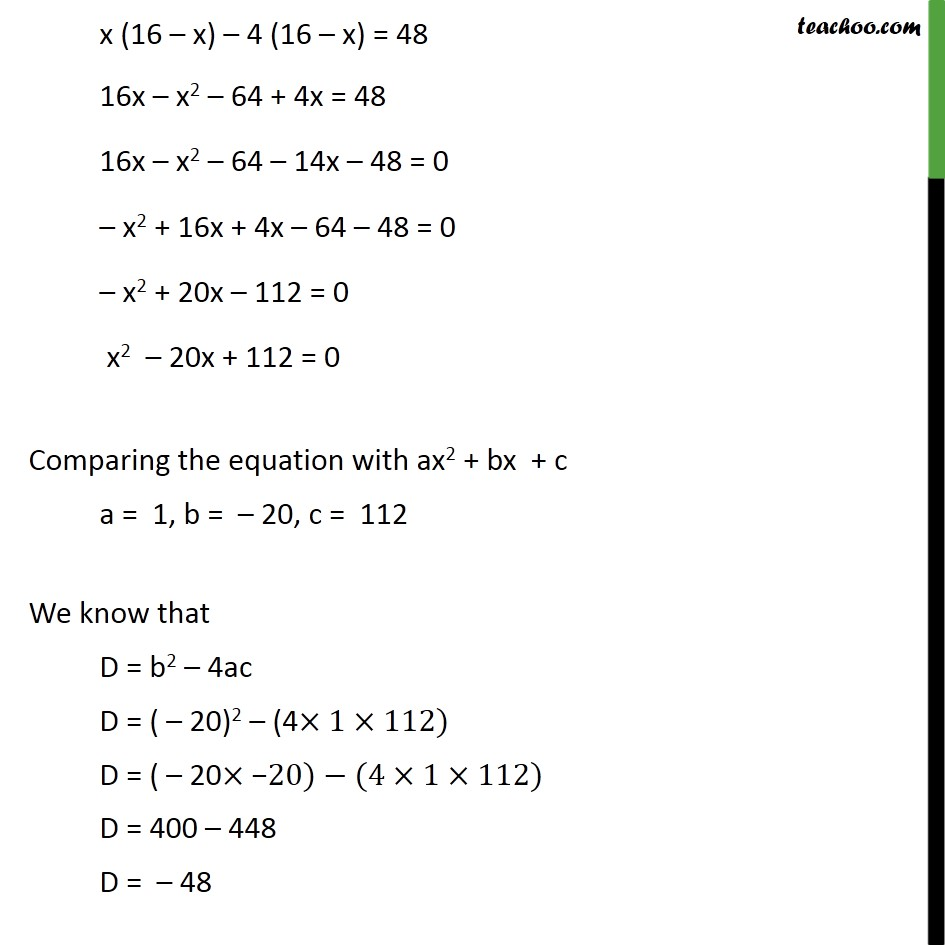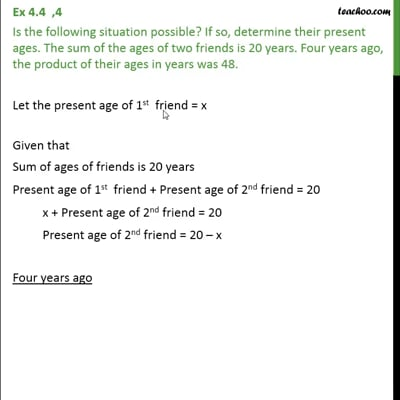Ex 4.4

Chapter 4 Class 10 Quadratic Equations
Serial order wiseThis video is only available for Teachoo black users

Get live Maths 1-on-1 Classs - Class 6 to 12

### Transcript

Ex 4.4 ,4 Is the following situation possible? If so, determine their present ages. The sum of the ages of two friends is 20 years. Four years ago, the product of their ages in years was 48. Let the present age of 1st friend = x Given that Sum of ages of friends is 20 years Present age of 1st friend + Present age of 2nd friend = 20 x + Present age of 2nd friend = 20 Present age of 2nd friend = 20 – x Four years ago Age of 1st friend = x – 4 Age of 2nd friend = (20 – x) – 4 = 16 – x Product of their ages 4 years ago was 48 (x – 4) (16 – x) = 48 x (16 – x) – 4 (16 – x) = 48 16x – x2 – 64 + 4x = 48 16x – x2 – 64 – 14x – 48 = 0 – x2 + 16x + 4x – 64 – 48 = 0 – x2 + 20x – 112 = 0 x2 – 20x + 112 = 0 Comparing the equation with ax2 + bx + c a = 1, b = – 20, c = 112 We know that D = b2 – 4ac D = ( – 20)2 – (4×1×112) D = ( – 20×"−" 20)−(4×1×112) D = 400 – 448 D = – 48 Since D < 0 The equation has no real roots Hence, the given situation is not possible